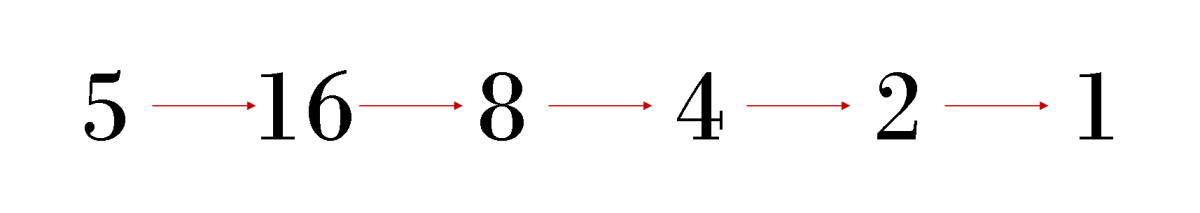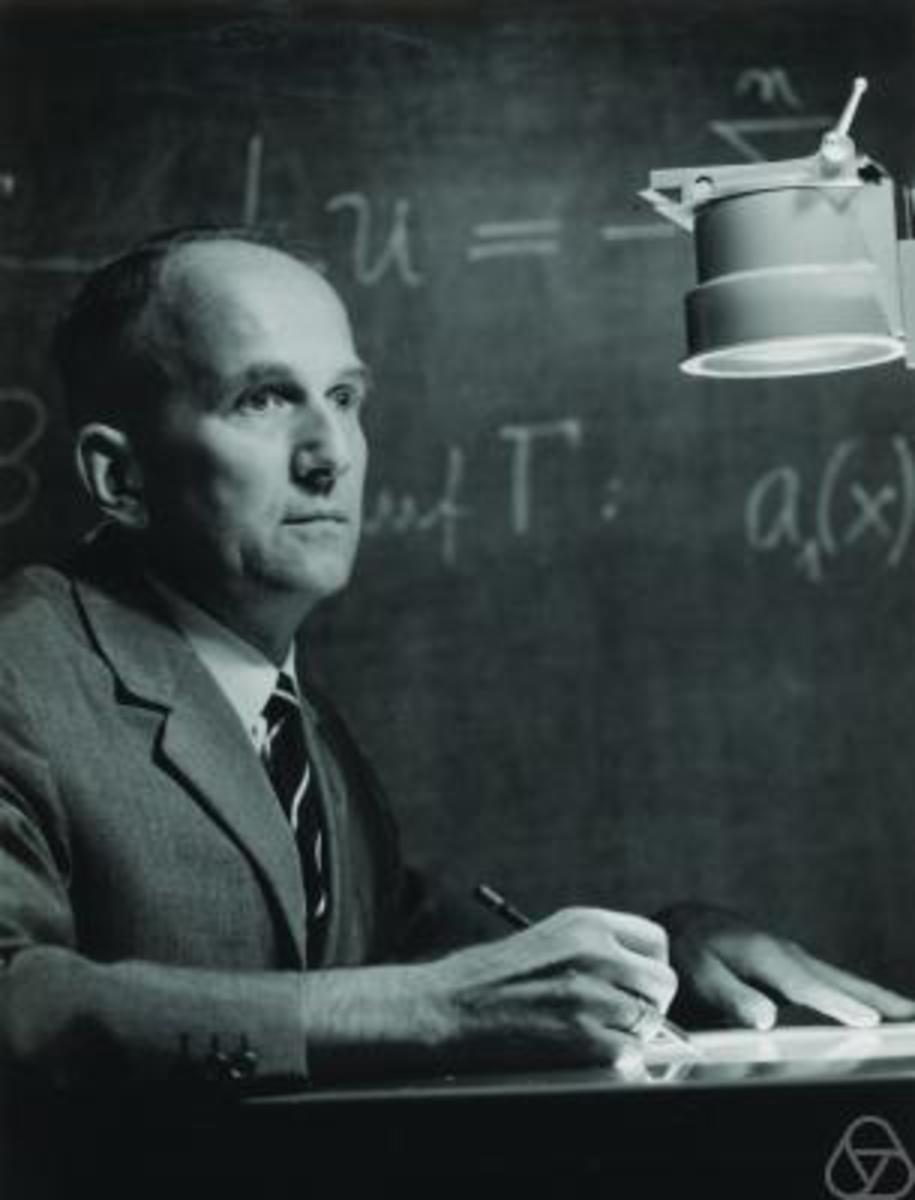# What Is the Collatz Conjecture? Unsolved Mathematical Problems

• Author:
• Updated date:

I am a former maths teacher and owner of DoingMaths. I love writing about maths, its applications, and fun mathematical facts.A Collatz Cycle starting at fiveOwn image

## The Collatz Conjecture

The Collatz conjecture is one of those pieces of mathematics which is easy to set-up and describe, but incredibly difficult to prove. So difficult in fact that many prominent mathematicians have tried and failed to prove that it works in all cases.

The Collatz conjecture is set up as follows:

1. Take any natural number (a positive whole number).

2. If your number is even, divide it by two. If your number is odd, multiply by three and then add one.

3. Take your answer and apply step 2 again.

4. Keep repeating this.

The Collatz conjecture states that whichever natural number you start with, by following these instructions you will always get to the number one.

## How Did the Collatz Conjecture Get Its Name?

The Collatz conjecture is named after the German mathematician, Lothar Collatz (1910 - 1990). As a professor at the Technical University of Hanover and then at the University of Hamburg, Collatz worked in numerical analysis and published many important papers in this area. However it is the conjecture bearing his name for which he is best remembered.Lothar Collatz (1910 - 1990), creator of the Collatz ConjectureKonrad Jacobs

## Stating the Collatz Conjecture Mathematically

We can describe the Collatz conjecture in a more mathematical way by using a piecewise function. This is a function f(x) which gives different results depending upon the values of x entered. As our function changes depending upon whether x is odd or even, we can write it as follows:

## Example 1

Let's try an example. We will start with the number 12.

12 is even, so f(12) = 12 ÷ 2 = 6

6 is also even, so f(6) = 6 ÷ 2 = 3

Scroll to Continue

## Read More From Owlcation

3 is odd, so f(3) = 3 × 3 + 1 = 10

Following on like this we get:

f(10) = 10 ÷ 2 = 5

f(5) = 3 × 5 + 1 = 16

f(16) = 16 ÷ 2 = 8

f(8) = 8 ÷ 2 = 4

f(4) = 4 ÷ 2 = 2

f(2) = 2 ÷ 2 = 1

We have reached 1 as the Collatz conjecture stated.

We can see the cycle of numbers we have gone through by displaying them together with arrows.

12 → 6 → 3 → 10 → 5 → 16 → 8 → 4 → 2 → 1

## Example 2

Let's try again with a different number, 19.

This time I will show only the path through the numbers.

19 → 58 → 29 → 88 → 44 → 22 → 11 → 34 → 17 → 52 → 26 → 13 → 40 → 20 → 10 → 5 → 16 → 8 → 4 → 2 → 1.

This one took us a bit longer, but again it eventually reached 1 as the conjecture stated it would.

## Interesting Facts About the Collatz Conjecture

1. A number will never appear twice in the sequence before you get to the number one.

If this happened, you would end up in a cycle constantly repeating the same numbers, hence never get to 1.

2. An odd number is always followed by an even number.

This is easily proved by considering how odd and even numbers are defined. If we write our odd number as 2m + 1 for some whole number m, applying the odd number rule for our conjecture gives us 3(2m + 1) + 1 = 6m + 4 = 2(3m +2). As this is a multiple of 2, it must be an even number.

3. The numbers 13 and 80 give the same cycle of results.

As 13 is odd, we multiply by 3 and add 1 to get 40. As 80 is even, we halve it to also get 40. As both cycles are now at 40, they must now follow the same cycle. 13 and 80 aren't the only numbers that have the same cycle of results. See how many others you can find.

## Does the Collatz Conjecture Work For Every Natural Number?

It seems strange for something that looks so simple, but the Collatz conjecture has never been proven to be true for all numbers.

People have searched for counter-examples and all the numbers up to 268 (approximately 2.95 × 1020) have been tested without anybody managing to find a number that doesn't work. But just like with all numerical problems, finding millions, or even billions or trillions of examples that work, doesn't mean that there isn't one out there that won't work.

For a number not to work, its sequence would need to either get stuck in a cycle that doesn't include the number 1, or keep on increasing forever. As mentioned above, no such number has yet been found.

Many mathematicians have worked on this problem and some have come close to a proof. For example, the Australian mathematician Terence Tao proved that for almost all numbers n, the sequence will eventually get below n/2, √n and ln n (as well as some other functions). In simpler terms, he proved that almost all numbers will reach a point that is as low as we want. While not quite a full proof, this is remarkably close.

Due to the work of Tao and other mathematicians who have come close to proofs and the sheer amount of numbers which have been positively tested, it is generally agreed that the Collatz Conjecture is true for all natural numbers. Maybe one day somebody will finally prove it completely.

## Sources and Further Reading

This content is accurate and true to the best of the author’s knowledge and is not meant to substitute for formal and individualized advice from a qualified professional.